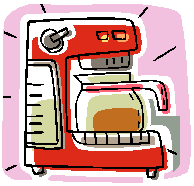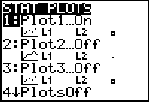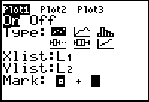Exponential Regression Model Example
(when doing an exponential regression, the y-values must be greater than 0)

Data:  The data at the right shows the cooling temperatures of a freshly brewed cup of coffee after it is poured from the brewing pot into a serving cup.  The brewing pot temperature is approximately 180º F.Time (mins)
Temp ( º F)
0
179.5
5
168.7
8
158.1
11
149.2
15
141.7
18
134.6
22
125.4
25
123.5
30
116.3
34
113.2
38
109.1
42
105.7
45
102.2
50
100.5
 Task: a.) Determine an exponential regression model equation to represent this data. b.) Graph the new equation. c.) Decide whether the new equation is a "good fit" to represent this data. d.) Based upon the new equation, what was the initial temperature of the coffee? e.) Interpolate data:  When is the coffee at a temperature of 106 degrees? f.) Extrapolate data:  What is the predicted temperature of the coffee after 1 hour? g.) In 1992, a woman sued McDonald's for serving coffee at a temperature of 180º that caused her to be severely burned when the coffee spilled.  An expert witness at the trial testified that liquids at 180º will cause a full thickness burn to human skin in two to seven seconds.  It was stated that had the coffee been served at 155º, the liquid would have cooled and avoided the serious burns.  The  woman was awarded over 2.7 million dollars.  As a result of this famous case, many restaurants now serve coffee at a temperature around 155º.   How long should restaurants wait (after pouring the coffee from the pot) before serving coffee, to ensure that the coffee is not hotter than 155º ? h.) If the temperature in the room is 76° F, what will happen to the temperature of the coffee, after being poured from the pot, over an extended period of time?

 Step 1.  Enter the data into the lists.  For basic entry of data, see Basic Commands.Step 2.  Create a scatter plot of the data.       Go to STATPLOT (2nd Y=) and choose the first plot.  Turn the plot ON, set the icon to Scatter Plot (the first one), set Xlist to L1 and Ylist to L2 (assuming that is where you stored the data), and select a Mark of your choice.Step 3.  Choose Exponential Regression Model.      Press STAT, arrow right to CALC, and arrow down to 0: ExpReg.  Hit ENTER.  When ExpReg appears on the home screen, type the parameters L1, L2, Y1.  The Y1 will put the equation into Y= for you.  (Y1 comes from VARS → YVARS, #Function, Y1)The exponential regression equation is(answer to part a) Step 4.  Graph the Exponential Regression Equation from Y1.      ZOOM #9 ZoomStat to see the graph.(answer to part b) Step 5.  Is this model a "good fit"?      The correlation coefficient, r, is -.9849556976 which places the correlation into the "strong" category.  (0.8 or greater is a "strong" correlation)      The coefficient of determination, r 2, is .9701377262 which means that 97% of the total variation in y can be explained by the relationship between x and y.       Yes, it is a very "good fit".          (answer to part c)Step 6.        Based upon the new equation, what was the initial temperature of the coffee? The exponential regression equation iswhere x stands for time.  The initial temperature would occur when the time equals zero.  Substituting zero for x gives an initial temperature of 171.462º.                           (answer to part d) Step 7.  Interpolate: (within the data)    When is the coffee at a temperature of 106 degrees?   Go to TBLSET (above WINDOW) and set the TblStart to 42 (since 42 minutes gives a temperature close to 106º).  Set the delta Tbl to a decimal setting of your choice.  Go to TABLE (above GRAPH) and arrow up or down to find your desired temp of 106º, in the Y1 column.(answer to part e: after approx. 40.7 minutes) Step 8. Extrapolate data: (beyond the data)      What is the predicted temperature of the coffee after 1 hour?     Change 1 hour to 60 minutes.  With your exponential equation in Y1, go to the home screen and type Y1(60).  Press ENTER.(answer to part f: 84.4º F) Step 9.   How long should the restaurants wait (after pouring the coffee from the pot) before serving coffee, to ensure that the coffee is not hotter than 155º ? Repeat procedure from Step 7:(answer to part g: approx. 8.5  minutes) Step 10.  If the temperature in the room is 76° F, what will happen to the temperature of the coffee, after being poured from the pot, over an extended period of time? The calculator's exponential equation shows that the coffee will reach room temperature after 68.8 minutes.  This exponential graph is asymptotic to the x-axis, implying that the y-values (degrees) approach zero.  This can be seen by observing successively larger values substituted into the calculator's exponential equation (see home screen substitutions below).  Even though the graph approaches zero asymptotically, we know that the temperature of the coffee will stop cooling once it reaches room temperature of 76º, and will not continue following the curve of the graph.NOTE: The temperature of the coffee stops cooling when it reaches the room temperature of 76°. The existence of the room temperature will actually affect the final exponential equation. Instead of the graph being asymptotic to the x-axis, the graph is actually asymptotic to the room temperature. If you visualize the room temperature as y = 76º, you will notice that there will be changes in the graph and consequently changes in the resulting equation. You can see how room temperature affects the graph and the equation for this problem at Newton's Law of Cooling.Finding Your Way Around TABLE of  CONTENTS